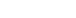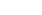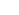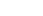How Cheenta works to ensure student success?
Explore the Back-Story

# Test of Mathematics Solution Subjective 125 - Function on Natural NumbersThis is a Test of Mathematics Solution Subjective 125  (from ISI Entrance). The book, Test of Mathematics at 10+2 Level is Published by East West Press. This problem book is indispensable for the preparation of I.S.I. B.Stat and B.Math Entrance.

## Problem

Letbe the function defined by f(0) = 0, f(1) = 1, and f(n) = f(n-1) + f(n-2), for, whereis the set of all non negative integers. Prove the following results:

• f(n) < f(n+1) for all• There exists precisely four non-negative integers n for which f(f(n)) = f(n).
• f(5n) is divisible by 5, for all n.

## Solution

This problem gives us an opportunity to discuss Fibonacci Sequence (which is defined by this function) and solving linear recursions. However none of these two discussions are necessary to solve the problem. Hence we leave those discussions for class and focus on the solution itself:

Clearly f(2) = 1, and f(3) = f(2) + f(1) = 2. Hence  f(3) is positive. We use strong form of induction and assume that up to n, all f(n) is positive.

Then f(n+1) = f(n) + f(n-1) .

Since f(n-1) is positive. Hence f(n+1) > f(n). This solves first part.

For second part we observe

• f(f(0)) = f(0)
• f(f(1)) = f(1) (as f(1) = 1)
• As f(2) = f(0) + f(1) = 1, we have f(f(2)) = f(1) = 1 = f(2).
• Next observe that
• f(3) = f(2) + f(1) = 1 + 1= 2
• f(4) = f(3) + f(2) = 2 + 1 = 3
• f(5) = f(4) + f(3) = 3 + 2 = 5
• So f(f(5)) = f(5) as f(5) = 5

So we got four numbers 0, 1, 2, 5, for which f(f(n)) = f(n) by observation. We wish to show that this does not happen for any value of n greater than 5.

Clearly, if f(f(n)) = f(n), then f(n) = n. We will show that f(n) > n for all n greater than 5. (If we can show that then it will automatically show that f(n) is not equal n for any value greater than 5, implying f(f(n)) not equal to f(n) for any other value apart from the ones that we have found earlier by trial and error).

Note that f(6) = f(5) + f(4) = 5 + 3 = 8. That is f(6) > 6. Similarly f(7) = f(6)+ f(5) = 8+5 = 13 implying f(7)> 7.

We will again use strong for of induction. Suppose f(k) > k for all values of k from 6 to n. Using this assumption we will show that f(n+1) > n+1.

f(n+1) = f(n) + f(n-1). By induction f(n) > n and f(n-1) > n-1. This implies f(n+1) > n + n -1 = 2n -1.

But 2n-1 > n+1 (as n > 2). Hence it follows that f(n+1) > n+1. This solves second part.

Finally we wish to show that 5 divides f(5n).

We again use induction. First note that f(5) = 5 hence divisible by 5. Assume that the claim is true for n-1. That is 5 divides f(5(n-1)). Using this assumption we wish to show that 5 divides f(5n). Now note that:

f(5n)

= f(5n-1) + f(5n-2)

= f(5n-2) + f(5n-3) + f(5n-3) + f(5n-4)

= f(5n-3) + f(5n-4) + f(5n- 4) + f(5n-5) + f(5n-4) + f(5n- 5) + f(5n - 4)

= f(5n-4) + f(5n-5) + f(5n-4) + f(5n- 4) + f(5n-5) + f(5n-4) + f(5n- 5) + f(5n - 4)

= 5 f(5n-4) + 3 f(5n-5)

Clearly 5f(5n-4) is divisible by 5. Also 3 f(5n-5) is divisible by 5 as by inductive assumption f(5n-5) is divisible by 5. Hence their sum 5 f(5n-4) + 3 f(5n-5) is divisible by 5. This shows 5 divides 5 divides f(5n).

This completes the proof of third part.

Actually the problem is more fun if we bring in ideas of fibonacci numbers and work with it's analytical form by solving the linear recurrence given here.

## Chatuspathi:

• What is this topic: Mathematical Induction
• What are some of the associated concept: Fibonacci Numbers, Linear Recurrences
• Where can learn these topics: I.S.I. & C.M.I.  Entrance course of Cheenta, discusses these topics in the ‘Number Theory’ module.
• Book Suggestions: Elementary Number Theory by David BurtonThis is a Test of Mathematics Solution Subjective 125  (from ISI Entrance). The book, Test of Mathematics at 10+2 Level is Published by East West Press. This problem book is indispensable for the preparation of I.S.I. B.Stat and B.Math Entrance.

## Problem

Letbe the function defined by f(0) = 0, f(1) = 1, and f(n) = f(n-1) + f(n-2), for, whereis the set of all non negative integers. Prove the following results:

• f(n) < f(n+1) for all• There exists precisely four non-negative integers n for which f(f(n)) = f(n).
• f(5n) is divisible by 5, for all n.

## Solution

This problem gives us an opportunity to discuss Fibonacci Sequence (which is defined by this function) and solving linear recursions. However none of these two discussions are necessary to solve the problem. Hence we leave those discussions for class and focus on the solution itself:

Clearly f(2) = 1, and f(3) = f(2) + f(1) = 2. Hence  f(3) is positive. We use strong form of induction and assume that up to n, all f(n) is positive.

Then f(n+1) = f(n) + f(n-1) .

Since f(n-1) is positive. Hence f(n+1) > f(n). This solves first part.

For second part we observe

• f(f(0)) = f(0)
• f(f(1)) = f(1) (as f(1) = 1)
• As f(2) = f(0) + f(1) = 1, we have f(f(2)) = f(1) = 1 = f(2).
• Next observe that
• f(3) = f(2) + f(1) = 1 + 1= 2
• f(4) = f(3) + f(2) = 2 + 1 = 3
• f(5) = f(4) + f(3) = 3 + 2 = 5
• So f(f(5)) = f(5) as f(5) = 5

So we got four numbers 0, 1, 2, 5, for which f(f(n)) = f(n) by observation. We wish to show that this does not happen for any value of n greater than 5.

Clearly, if f(f(n)) = f(n), then f(n) = n. We will show that f(n) > n for all n greater than 5. (If we can show that then it will automatically show that f(n) is not equal n for any value greater than 5, implying f(f(n)) not equal to f(n) for any other value apart from the ones that we have found earlier by trial and error).

Note that f(6) = f(5) + f(4) = 5 + 3 = 8. That is f(6) > 6. Similarly f(7) = f(6)+ f(5) = 8+5 = 13 implying f(7)> 7.

We will again use strong for of induction. Suppose f(k) > k for all values of k from 6 to n. Using this assumption we will show that f(n+1) > n+1.

f(n+1) = f(n) + f(n-1). By induction f(n) > n and f(n-1) > n-1. This implies f(n+1) > n + n -1 = 2n -1.

But 2n-1 > n+1 (as n > 2). Hence it follows that f(n+1) > n+1. This solves second part.

Finally we wish to show that 5 divides f(5n).

We again use induction. First note that f(5) = 5 hence divisible by 5. Assume that the claim is true for n-1. That is 5 divides f(5(n-1)). Using this assumption we wish to show that 5 divides f(5n). Now note that:

f(5n)

= f(5n-1) + f(5n-2)

= f(5n-2) + f(5n-3) + f(5n-3) + f(5n-4)

= f(5n-3) + f(5n-4) + f(5n- 4) + f(5n-5) + f(5n-4) + f(5n- 5) + f(5n - 4)

= f(5n-4) + f(5n-5) + f(5n-4) + f(5n- 4) + f(5n-5) + f(5n-4) + f(5n- 5) + f(5n - 4)

= 5 f(5n-4) + 3 f(5n-5)

Clearly 5f(5n-4) is divisible by 5. Also 3 f(5n-5) is divisible by 5 as by inductive assumption f(5n-5) is divisible by 5. Hence their sum 5 f(5n-4) + 3 f(5n-5) is divisible by 5. This shows 5 divides 5 divides f(5n).

This completes the proof of third part.

Actually the problem is more fun if we bring in ideas of fibonacci numbers and work with it's analytical form by solving the linear recurrence given here.

## Chatuspathi:

• What is this topic: Mathematical Induction
• What are some of the associated concept: Fibonacci Numbers, Linear Recurrences
• Where can learn these topics: I.S.I. & C.M.I.  Entrance course of Cheenta, discusses these topics in the ‘Number Theory’ module.
• Book Suggestions: Elementary Number Theory by David Burton

### Knowledge Partner